# DAV Class 5 Maths Chapter 4 Worksheet 1 Solutions

The DAV Class 5 Maths Book Solutions Pdf and DAV Class 5 Maths Chapter 4 Worksheet 1 Solutions of Fractional Numbers offer comprehensive answers to textbook questions.

## DAV Class 5 Maths Ch 4 Worksheet 1 Solutions

Question 1.
Encircle the fraction in its lowest term. The first one is done for you.
(a) $$\frac{2}{5}, \frac{4}{10}, \frac{8}{20}, \frac{6}{15}$$
Solution:(b) $$\frac{4}{24}, \frac{6}{36}, \frac{1}{6}, \frac{3}{18}$$
Solution:(c) $$\frac{5}{15}, \frac{2}{6}, \frac{4}{12}, \frac{1}{3}$$
Solution:(d) $$\frac{4}{5}, \frac{12}{15}, \frac{32}{40}, \frac{16}{20}$$
Solution: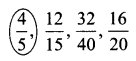(e) $$\frac{6}{10}, \frac{24}{40}, \frac{3}{5}, \frac{18}{30}$$
Solution: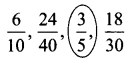Question 2.
Tick (✓) those fractions which are in the lowest term.
(a) $$\frac{6}{10}$$
Solution:

(b) $$\frac{5}{32}$$
Solution: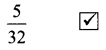(c) $$\frac{1}{8}$$
Solution:(d) $$\frac{5}{8}$$
Solution: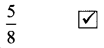(e) $$\frac{13}{15}$$
Solution: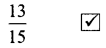(f) $$\frac{81}{90}$$
Solution:

(g) $$\frac{21}{15}$$
Solution:

(h) $$\frac{26}{42}$$
Solution:Question 3.
Reduce into the lowest term.
(a) $$\frac{9}{12}$$
Solution:
$$\frac{9}{12}$$
HCF of 9 and 12 is 3.
$$\frac{9}{12} \div \frac{3}{3}=\frac{3}{4}$$ is the lowest term.

(b) $$\frac{6}{20}$$
Solution:
$$\frac{6}{20}$$
HCF of 6 and 20 is 2
$$\frac{6}{20} \div \frac{2}{2}=\frac{3}{10}$$ is the lowest term.

(c) $$\frac{10}{22}$$
Solution:
HCF of 10 and 22 is 2
$$\frac{10}{22} \div \frac{2}{2}=\frac{5}{11}$$ is the lowest term.

(d) $$\frac{18}{24}$$
Solution:
HCF of 18 and 24 is 6
$$\frac{18}{24} \div \frac{6}{6}=\frac{3}{4}$$ is the lowest term.

(e) $$\frac{28}{56}$$
Solution:
HCF of 28 and 56 is 28.
$$\frac{28}{56} \div \frac{28}{28}=\frac{1}{2}$$ is the lowest term.

(f) $$\frac{12}{60}$$
Solution:
HCF of 12 and 60 is 12.
$$\frac{12}{60} \div \frac{12}{12}=\frac{1}{5}$$ is the lowest term.

(g) $$\frac{15}{45}$$
Solution:
HCF of 15 and 45 is 15.
$$\frac{15}{45} \div \frac{15}{15}=\frac{1}{3}$$ is the lowest term.

(h) $$\frac{48}{54}$$
Solution:
The HCF of 48 and 54 is 6.
$$\frac{48}{54} \div \frac{6}{6}=\frac{8}{9}$$ is the lowest term.(i) $$\frac{36}{48}$$
Solution:
The HCF of 36 and 48 is 12.
$$\frac{36}{48} \div \frac{12}{12}=\frac{3}{4}$$ is the lowest term.

(j) $$\frac{22}{55}$$
Solution:
The HCF of 22 and 55 is 11.
$$\frac{22}{55} \div \frac{11}{11}=\frac{2}{5}$$ is the lowest term.

Review Exercise

Question 1.
Write the next three equivalent fractions.
(i) $$\frac{2}{6}, \frac{4}{12}, \frac{6}{18}$$, ____, _____, _____
Solution:
$$\frac{8}{24}, \frac{10}{30}, \frac{12}{36}$$

(ii) $$\frac{1}{7}, \frac{2}{14}, \frac{3}{21}$$, ____, _____, _____
Solution:
$$\frac{4}{28}, \frac{5}{35}, \frac{6}{42}$$

(iii) $$\frac{5}{9}, \frac{10}{18}, \frac{15}{27}$$, ____, _____, _____
Solution:
$$\frac{20}{36}, \frac{25}{45}, \frac{30}{54}$$

Question 2.
Convert the following into improper fractions. The first is done for you.
(a) $$3 \frac{1}{5}$$ = __________
Solution:
$$3 \frac{1}{5}$$ = $$\frac{16}{5}\left(\frac{(3 \times 5)+1}{5}\right)$$

(b) $$5 \frac{1}{7}$$ = __________
Solution:
$$5 \frac{1}{7}$$ = $$\frac{36}{7}\left(\frac{7 \times 5+1}{7}\right)$$

(c) $$33 \frac{1}{3}$$ = _________
Solution:
$$33 \frac{1}{3}$$ = $$\frac{100}{3}\left(\frac{33 \times 3+1}{3}\right)$$

(d) $$2 \frac{2}{9}$$ = __________
Solution:
$$2 \frac{2}{9}$$ = $$\frac{20}{9}\left(\frac{2 \times 9+2}{9}\right)$$Question 3.
Convert the following into a mixed number. The first one is done for you.
(i) $$\frac{50}{7}$$ = ________
Solution: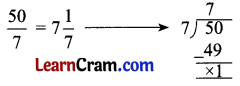(ii) $$\frac{11}{9}$$ = ________
Solution:(iii) $$\frac{23}{8}$$ = ________
Solution:(iv) $$\frac{78}{17}$$ = ________
Solution: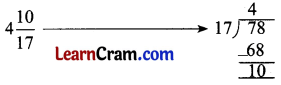Question 4.
Fill in the blanks.
(a) Fractions having the same denominators are called _______ fractions.
Solution:
like

(b) A fractional number whose numerator is more significant than its denominator is called an _________ fraction.
Solution:
improper

(c) $$\frac{1}{2}, \frac{1}{7}, \frac{1}{11}, \frac{1}{4}$$ and $$\frac{1}{3}$$ are called ________ fractions.
Solution:
unit

(d) $$3 \frac{1}{8}$$ is a _______ number.
Solution:
mixed

(e)Solution:
64

DAV Class 5 Maths Chapter 4 Worksheet 1 Notes

Equivalent Fractions:
Fractions which express the value of the same part of a whole are called Equivalent Fractions.
e.g., $$\frac{1}{2}, \frac{2}{4}, \frac{4}{8}, \frac{8}{16}$$

Improper Fractions:
Fractions whose numbers are greater than denominators are called Improper Fractions.
e.g., $$\frac{4}{2}, \frac{7}{3}, \frac{9}{2}$$

Proper Fractions:
Fractions whose numerators are smaller than denominators are called Proper Fractions.
e.g., $$\frac{2}{7}, \frac{5}{9}, \frac{3}{11}$$

Like Fractions:
Fractions having the same denominators are called Like Fractions.
e.g., $$\frac{3}{7}, \frac{2}{7}, \frac{8}{7}$$Unlike Fractions:
Fractions having different denominators are called Unlike Fractions.
e.g., $$\frac{3}{5}, \frac{3}{13}, \frac{7}{9}$$

Unit Fractions:
Fractions whose numerators are equal to one are called Unit Fractions.
e.g., $$\frac{1}{7}, \frac{1}{9}, \frac{1}{15}$$

Mixed Number: An improper fraction written as a combination of a whole and a proper fraction is called a Mixed Number.
e.g., $$2 \frac{1}{9}, 3 \frac{1}{7}, 1 \frac{1}{3}$$

To reduce a fraction into the lowest terms, we go on dividing the numerator and denominator by their common factors till we are left with a fraction having 1 as the only common factor of both its numerator and denominator.
e.g., $$\frac{4}{9}=\frac{2}{3}$$ (lowest term)

To get the product of a whole number and a fractional number, we multiply the whole number and numerator of the fractional number. The denominator remains the same.
e.g., $$8 \times \frac{2}{3}=\frac{8 \times 2}{3}=\frac{16}{3}$$

To get the product of two fractional numbers, we multiply the numerators and the denominators separately.
e.g., $$\frac{2}{5} \times \frac{3}{5}=\frac{2 \times 3}{5 \times 5}=\frac{6}{25}$$

By interchanging the numerator and the denominator of a fractional number, we get the reciprocal of that number.
e.g., $$\frac{4}{5}=\frac{5}{4}$$ (reciprocal number)

When we divide a fractional number with a whole number or with another fractional number, we first change the division sign to the multiplication sign and multiply by the reciprocal of the divisor.
e.g., (i) $$4 \div \frac{2}{3}=4 \times \frac{3}{2}=\frac{12}{2}$$ or 6
(ii) $$\frac{4}{2} \div \frac{3}{2}=\frac{4}{2} \times \frac{2}{3}=\frac{8}{6}=\frac{4}{3}$$

Fractions in the Lowest Terms
Let us take a fraction $$\frac{5}{3}$$
(a) $$\frac{5}{3} \times \frac{2}{2}=\frac{10}{6}$$
Numerator and Denominator are called terms of a fraction.

(b) $$\frac{5}{3} \times \frac{3}{3}=\frac{15}{9}$$
When we multiply the numerator and denominator of a fraction by a common number, we get higher terms of the fraction.(c) $$\frac{6}{8} \div \frac{2}{2}=\frac{3}{4}$$
(d) $$\frac{10}{12} \div \frac{2}{2}=\frac{5}{6}$$
When we divide the numerator and denominator of a fraction by a common factor other than 1, we get lower terms of the fraction.

We cannot further divide $$\frac{3}{7}$$ by a common number. It can be divided only by the common factor 1.
$$\frac{3}{7} \div \frac{1}{1}=\frac{3}{7}$$
So $$\frac{3}{7}$$ is in the lowest terms.

In order to reduce a fraction into the lowest term, we go on dividing the numerator and denominator by their common factors till we are left with a fraction having 1 as the only common factor.

Example 1.
Reduce $$\frac{15}{25}$$ in its lowest term.
Solution:
$$\frac{15}{25} \div \frac{5}{5}=\frac{3}{5}$$ is in the lowest term.
Dividing by common factor 2. (because 3 and 5 have no common factor other than 1)

Simplification of Fractions by using HCF

Example 1.
Let us reduce $$\frac{18}{20}$$ to the lowest term.
Solution:
HCF of 18 and 20 is 2.
$$\frac{18}{20} \div \frac{2}{2}$$ (Divided by HCF)
$$\frac{9}{10}$$ (is the lowest term)